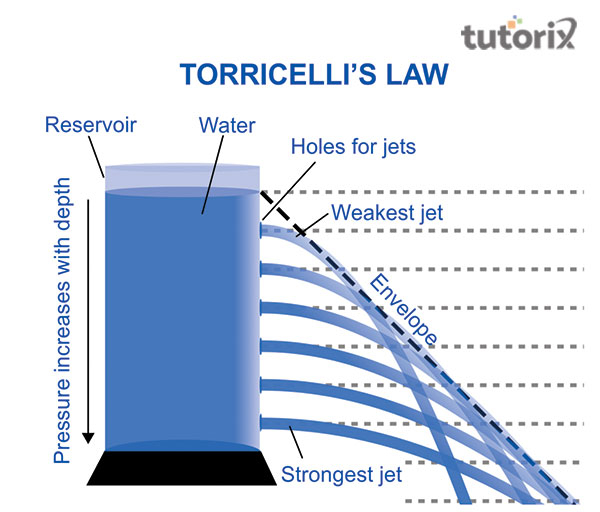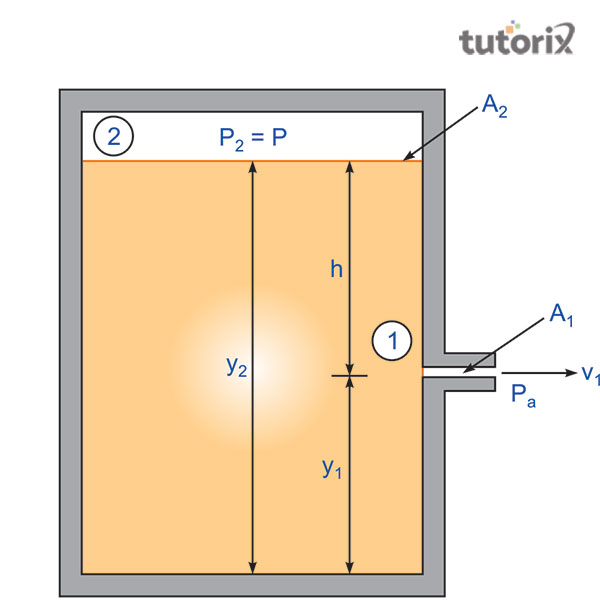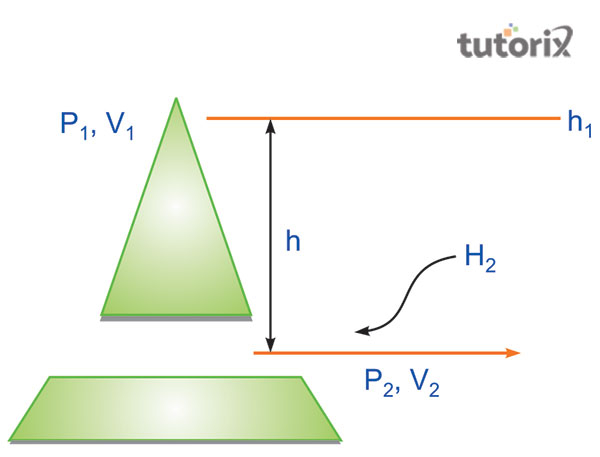# Torricellis Law

Torricelli’s law denotes that the flow speed of the fluid is equivalent to the speed of the object that is freely falling from a specific distance that is also equivalent to the height of the free surface of the fluid. Evangelista Torricelli was an Italian scientist who discovered the law in 1643. Later this law was shown as a specification of the principle of Bernoulli. This principle includes that the fluid flows through a steady system, and the density of a fluid is constant which refers to that, the fluid can be incompressible.

## What is the law of Torricelli?

The law of Torricelli of the theorem of Torricelli is derived for a particular condition. The conditions are, that the orifice should be small, and the velocity along with other losses should be ignored (Gao & Hu, 2020). In the case of the fluid flowing through a tiny orifice then the fluid velocity at the end is neglected in the equation of Bernoulli. From the law of Torricelli, it is derived that the efflux speed is not al all dependent on the flow direction of the fluid. In this case, the speed of the efflux flows through an orifice that can be presented by the formula of v = √2gh (Gao & Hu, 2020). The law of Torricelli can be commonly seen in daily life in several cases. For example, application of Torricelli’s law can be observed during overflowing of water from tank.

## Theorem of Torricelli

The physical law of Torricelli denotes that there is a major relationship between the height and velocity of the water tank. This law or equation explains that there is a relationship between the fluid that flows through a hole and the height of liquid in the liquid tank.Figure 1: The application of Torricelli’s law

The law of Torricelli is also known as Torricelli’s principle that mainly states the speed of fluids that are denoted by v. The flowing fluid that comes out of an orifice with the gravitational force in the tank is equally proportional to the square root of the vertical distance that is denoted by h (Williams, 2021). It can be said that a fluid efflux velocity of the orifice is equivalent to the falling height of a falling object that comes under the gravitational force.

## Law derivation of TorricelliFigure 2: The mechanical properties of Torricelli’s law

In this figure, A1 refers to the slit area and V1 stands for the velocity that helps the fluid to flow. A2 represents the area of the free surface of the fluid in the tank and the V2 denotes the fluid velocity of the free surface (Alvaro Berlanga, 2021). In the AV is constant then A1V1 is equivalent to A2V2. In the context of the figure, A2 > A1, the mechanical properties of this particular law depend on the formula Pa+ (1/2) ρ v12+ ρgy1= P+ρgy2 where ρ stands for the density of the fluid. V stands for the flowing speed of the liquid, and g denotes the gravitational acceleration that is applied to the flowing fluid. In this formula, y refers to the height of the fluid of the free surface.

## Application of Torricelli’s lawFigure 3: Application of Torricelli’s law

The application of Torricelli’s law is commonly seen in our daily life experiences. The application of this law is can be seen in during the overflow of water from the tank. Besides this, in the water dam, when the water is released from the dam application of this particular law can be seen (Gomez-Rojas et al. 2021). The direction of the velocity of the fluid is commonly seen. The application of Torricelli’s law is also involved in the simplified conditions in the context of the constant height as well as constant pressure. In the working process of fire removal cars, the law of Torricelli is also seen.

## Conclusion

This particular relationship can be stated in a different way with the formula

v²/2 + gh p/ρ = constant

In this formula, v denotes the flowing speed of the liquid, and g stands for the gravitational acceleration that is applied to the fluid. In the formula, h represents the height of the fluid that is over the reference point, ρ stands for the density of the fluid and P is equivalent to the atmospheric pressure that is mainly observed at the top level of the liquid container. In this formula, v is equivalent to 0 because the surface of the liquid that drops in height is compared to the speed of the fluid of the tank. Here, h can be 0 which is equivalent to p in a specific point of the atmosphere.

## FAQs

Q.1 What are the mechanical properties of Torricelli’s law?

The mechanical properties of the law are Pa+ (1/2) ρ v12+ ρgy1= P+ρgy2. In the formula, ρ denotes the density and V is speed.

Q.2 What is the equation of Torricelli’s law?

The equation of Torricelli’s law is Vfx2 = Vix2 + 2axΔx, where the velocity of the flowing fluids is dependent on the speed and the height of the fluid in the water tank. The constant pressure as well as the gravitational acceleration has a great influence on the velocity.

Q.3 What is the actual value of height in the law of Torricelli?

In the law of Torricelli, the value of height is equivalent to zero. The atmospheric pressure also influences the height of the fluid.

Q.4 What is the formula of Torricelli?

The formula of this law is v²/2 + gh p/ρ = constant. In the formula, ρ denotes the density and V is speed.